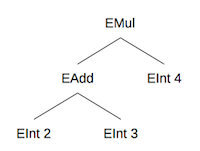# Module 12 Project

## Introduction

In this PA you will implement some analysis routines over a data structure called an expression tree. You will implement similar projects in Ruby, Haskell, and Prolog, and the goal is to explore the differences between the three languages.

In Ruby, you will extend existing Ruby classes to practice dynamic object-oriented programming. In Haskell, you will write pattern-matching routines to practice functional programming. In Prolog, you will write rules to practice logical programming.

Project starter files: `p4.zip`

## Requirements

You may ignore `Lexer.hs` and `Parser.hs`. They were auto-generated from grammar specification files using utilities called Alex and Happy, and they provide the backend code for parsing simple mathematical expressions. Here is a context-free grammar describing the language that this parser can recognize:

``````    E -> <int>
| E + E
| E - E
| E * E
| ( E )
``````

You may also ignore the definition of `Token` in `Defs.hs`, although you will need to use the definition of the `Expr` data type:

``````data Expr
= EInt Int
| EAdd Expr Expr
| ESub Expr Expr
| EMul Expr Expr
deriving (Show,Eq)
``````

This data type represents nodes in the expression parse tree. For instance, the expression `(2+3)*4` will be parsed into the following tree:`EInt`, `EAdd`, `ESub`, and `EMul` are all data value constructors, used to build or pattern match against an expression tree. `EInt` represents an integer terminal node, while the other three represent non-terminal arithmetic operation nodes. The last line of the `Expr` definition specifies that we wish to be able to convert the expressions to strings and compare them using Haskell built-in recursive definitions.

Using these value constructors, we could represent the parse tree shown above with the following Haskell expression:

``````EMul (EAdd (EInt 2) (EInt 3)) (EInt 4)
``````

Your task in this PA will be to write various functions to analyze expression trees. You will do this by writing functions that recurse over an expression tree based on data patterns.

You can see an example of a pattern-based function in `formatExpr` in `Defs.hs`. This function takes a tree and matches it against one of the four different patterns possible using the value constructors, returning a different form of string for each different pattern.

Most of the functions you write for this PA will have this general form. The function’s input will be an expression tree, and you will specify the output of the function for each pattern that the input could be matched against. You will likely want to make recursive calls in the non-terminal cases.

Below are descriptions of the functions you need to implement. You can also look at the test cases (in `Test.hs`) to see what they are supposed to do. You should think about what the definition of each function means for each `Expr` pattern. Unlike in the Ruby implementation, there are no “abstract” classes and so you will need to write patterns for each value constructor.

• `eval :: Expr -> Int` - Calculates the result of evaluating the mathematical expression. All operations should be done using integer arithmetic.
• `countOps :: Expr -> Int` - Counts the total number of arithmetic operations in an expression. All three operations (addition, subtraction, and multiplication) count for this process.
• `height :: Expr -> Int` - Calculates the height of the expression tree, where the height of a single-node tree is defined as being 1.
• `postfix :: Expr -> String` - Returns a flattened postfix string representation. For example, `(2+3)*4` in postfix notation would be ```"2 3 + 4 *"```. You should use the `show` function to convert integers to strings.
• `uniqInts :: Expr -> [Int]` - Extract a sorted list of all unique integers in an expression. Each integer should appear in the resulting list only once.

For the last function (`uniqInts`), you may find it helpful to write two helper functions:

• `uniq :: Eq t => [t] -> [t]` - Returns a copy of the given list with duplicates removed.
• `sort :: Ord t => [t] -> [t]` - Returns a sorted copy of the given list.

Some test routines are included in `Test.hs`. However, your submission will be graded based on additional tests not provided to you, so you should write your own test cases as well. You can also test your routines via the provided REPL (read/eval/print loop) by running `Main.hs`.

For this assignment, you may NOT use any classes or methods that are not in the Haskell Prelude. If you are unsure about a particular class or method, you can consult the documentation.

## Submission

Your program must contain implementations as described above. You must name your Haskell script file `P4.hs`. You must put your name in a comment at the top of the file. As usual, decompose your methods to make them more readable, use good names, indent properly, and so on. You will partly be graded on the readability of your code but mostly on whether it works.

Submit ONLY `P4.hs` to the appropriate assignment on Canvas by the deadline.